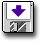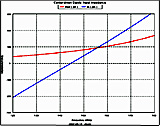Modeling a Center-driven Dipole with FEKO

 Geometry and setup Geometry: L= 1 m, a = 0.5 mm Mesh: Global mesh size: Edge length=40 mm, Segment length=0.2 mm, wire radius=0.01 mm Disable volume meshing Small features: default Advanced: Enable mesh smoothing Solution Frequency: Linearly spaced discrete points:50 MHz - 400 MHz, number of frequencies = 71 Excitations: Voltage source (1 V, 50 ohms)feko_dipole.zip Simulation result Simulation Time: 1 min 13 secs Number of metallic triangles: 328 Number of metallic edges (MOM): 3 Number of basis functions (MOM): 496 Decisions the user must make that affect the accuracy of the result The segment length should be smaller than a tenth of the free space wavelength. Segments should not be too short relative to the wire radius. Ideally, the segment length should be at least four times the radius. For MOM mesh elements, the side length of triangles should be shorter than approximately λ/5. If the memory constraints allow it, an edge length less than λ/10 is preferred. In this case, the edge length is λ/50. Comments Adding the source requires a gap between the two cylinders. What is the source gap length? In this model, the interval between two antenna parts is 0.4 mm. What meshing rules should be adhered to in this model? In FEKO, a segment is defined as a short section of wire (short in comparison with the wavelength). In this model, the voltage source is implemented using a short wire which connects the upper and the lower part of the antenna. Since the segment current flows only in the axial direction, the segments should not too short relative to the wire radius. Ideally, the segment length should be at least four times the radius. In this model, the wire radius is 0.01 mm. The "segment length" field in the Create Mesh dialog is set to 0.2. Another meshing guideline in FEKO is to ensure that the edge length of surface mesh elements is less than λ\10. This half-wave dipole resonates at about 150 MHz corresponding to a wavelength of about 2 m. In this model, the edge length is about λ/50. How can we simplify the round wire model? Using the equivalent radius concept, a round wire antenna can be approximated by a planar strip, or flat ribbon. The equivalent width of the ribbon is 4 times the round wire radius. Furthermore, since the radius of the dipole is much smaller than its length, the dipole can be also modeled as a vanishingly thin wire. Both the flat ribbon and the wire model significantly reduce the simulation time. More information...
 Screen shotsFig. 4. Input impedance at the first resonant frequency